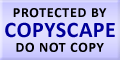Submit Assignment From Here
Name
:
Email
:
Phone
:
Country
:
Willing to pay US\$
:
Subject
:
Topic
:
Levels
:
:Hours
:

# Probability Homework Help

Probability is an estimate of how favorable it is for a certain event to occur. The values can be from 0% to 100% or from 0 to 1. Where, 0 is the chance of event not happening at all and 1 is the chance of event happening with surety. The higher degree of probability is more likely the outcome of the event. The lower the probability the lesser are chances of the event to happen.
The concept of probability is a new concept. The main reason for development of probability is gambling. We can say that the game of gambling is the impetus for the occurrence of study of probability. The theory of probability is the presentation of probable concepts in the terms that can have distinct meaning from their actual meanings. These probable terms have their basis from the mathematical rules and interpretations.

Probability Homework Help

The two most important events that occurred to formalize probability were Kolmogorov formulation and Cox formulation. Kolmogorov formulation is events which get interpretations and probability is used as a measure for the sets. Cox formulation probability is taken as it is, it is not analyzed the main pressure is on the construction of the propositions.

 Click here to submit assignment Statistics Homework Help Confidence Interval Estimation Continuous Probability Distribution Methods of Least Squares and Curve Fitting Estimation Theory Numerical Measures Principles of Data Reduction Properties of Random Sample

### Live Online Tutoring### Make Payment OnlineUS